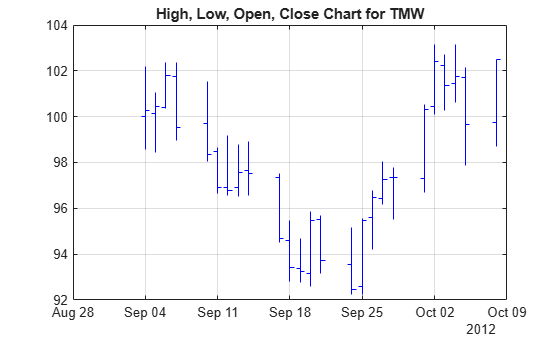Documentation

# highlow

High, low, open, close chart

`highlow` is updated to accept data input as a matrix, `timetable`, or `table`.

## Syntax

``highlow(Data)``
``highlow(Data,Color)``
``h = highlow(ax,___)``

## Description

example

````highlow(Data)` displays a highlow chart from a series of opening, high, low, and closing prices of a security. The plots are vertical lines whose top is the high, bottom is the low, open is a left tick, and close is a right tick. ```

example

````highlow(Data,Color)` adds an optional argument for `Color`. ```

example

````h = highlow(ax,___)` adds an optional argument for `ax`. ```

## Examples

collapse all

Load the file `SimulatedStock.mat`, which provides a timetable (`TMW`) for financial data for TMW stock. The highlow chart plots the price data using blue lines.

```load SimulatedStock.mat range = 1:25; highlow(TMW(range,:),'b'); title('High, Low, Open, Close Chart for TMW')```## Input Arguments

collapse all

Data for opening, high, low, and closing prices, specified as a matrix, table, or timetable. For matrix input, `Data` is an `M`-by-`4` matrix of opening, high, low, and closing prices stored in the corresponding columns. Timetables and tables with `M` rows must contain variables named `'Open'`, `'High'`, `'Low'`, and `'Close'` (case insensitive).

Data Types: `double` | `table` | `timetable`

(Optional) Three element color vector, specified as a ```[R G B]``` color vector or a string specifying the color name. The default color differs depending on the background color of the figure window. For more information, see `colorspec`.

Data Types: `double` | `string`

(Optional) Valid axis object, specified as an axes object. The highlow plot is created in the axes specified by `ax` instead of in the current axes (`ax = gca`). The option `ax` can precede any of the input argument combinations.

Data Types: `object`

## Output Arguments

collapse all

Graphic handle of the figure, returned as a handle object.